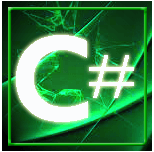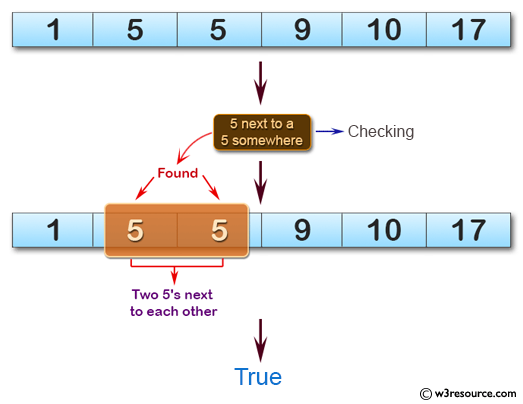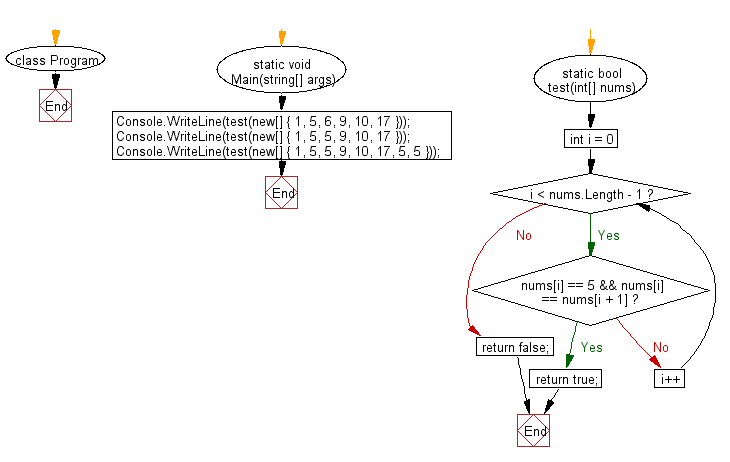﻿ C# Sharp exercises: Check if a given array of integers contains 5 next to a 5 somewhere - w3resource# C# Sharp Basic Algorithm Exercises: Check if a given array of integers contains 5 next to a 5 somewhere

## C# Sharp Basic Algorithm: Exercise-113 with Solution

Write a C# Sharp program to check if a given array of integers contains 5 next to a 5 somewhere.

Pictorial Presentation:Sample Solution:

C# Sharp Code:

``````using System;
namespace exercises
{
class Program
{
static void Main(string[] args)
{
Console.WriteLine(test(new[] { 1, 5, 6, 9, 10, 17 }));
Console.WriteLine(test(new[] { 1, 5, 5, 9, 10, 17 }));
Console.WriteLine(test(new[] { 1, 5, 5, 9, 10, 17, 5, 5 }));
}
static bool test(int[] nums)
{
for (int i = 0; i < nums.Length - 1; i++)
{
if (nums[i] == 5 && nums[i] == nums[i + 1]) return true;
}

return false;
}
}
}
```
```

Sample Output:

```False
True
True```

Flowchart:C# Sharp Code Editor:

Improve this sample solution and post your code through Disqus

What is the difficulty level of this exercise?

﻿

New Content: Composer: Dependency manager for PHP, R Programming